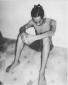# A reservoir is in the form of frustum of a cone, 68 ft. across the top, 35 ft. across the bottom, and 18 ft. deep. Find the cost

2 posts / 0 new
Renz Kolin Gaba...A reservoir is in the form of frustum of a cone, 68 ft. across the top, 35 ft. across the bottom, and 18 ft. deep. Find the cost
fitzmerl duronTo answer the question above, kailangan muna natin ng figure....It looks like this:Assuming that your reservoir is a closed tank, your reservoir looks ugly because it will be lined/covered with tiles...hehehehe...

Your reservoir is shaped in a frustrum of a cone and it is lined with tiles, we need to get the surface area of the frustrum of a cone.The surface area of the frustrum of a cone is...

$$Surface \space area = \pi (r_1^2 + r_2^2 + r_1r_2\sqrt{(r_1^2 - r_2^2)^2 + h^2})$$

where $r_1$ is radius of upper base of the frustrum of a cone, $r_2$ is radius of lower base of the frustrum of a cone, and $h$ is the height of the frustrum of a cone.

With that in mind, the surface area of the frustrum of a cone would be...

$$Surface \space area = \pi (r_1^2 + r_2^2 + r_1r_2\sqrt{(r_1^2 - r_2^2)^2 + h^2})$$ $$Surface \space area = \pi ((17.5 \space ft)^2 + (34 \space ft)^2 + (17.5 \space ft)(34 \space ft)\sqrt{((17.5 \space ft)^2 - (34 \space ft)^2)^2 + (18 \space feet)^2})$$ $$Surface \space area = 50237.51 ft^2$$

The cost of lining the reservoir with tiles then is $$50237.51 ft^2 \left( \right) = \color{green}{\ 50237.51}$$

and that is one expensive reservoir....

Alternate solutions are highly encouraged...

• Mathematics inside the configured delimiters is rendered by MathJax. The default math delimiters are $$...$$ and $...$ for displayed mathematics, and $...$ and $...$ for in-line mathematics.